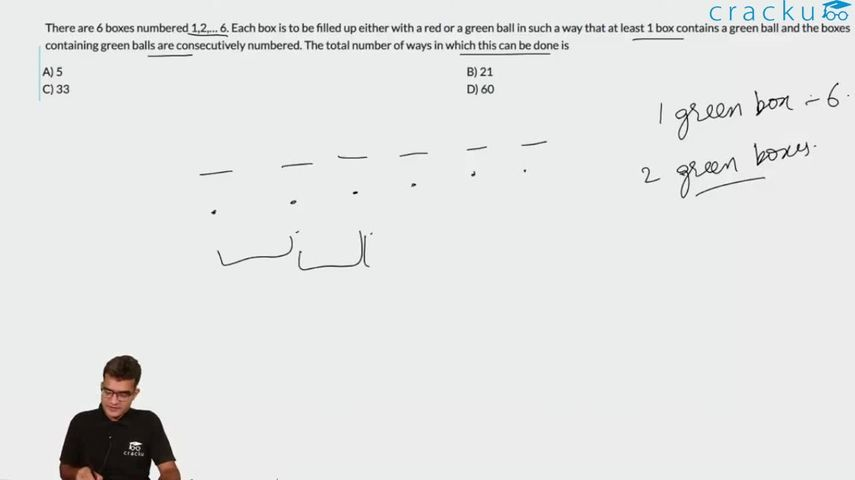Question 91

# There are 6 boxes numbered 1,2,… 6. Each box is to be filled up either with a red or a green ball in such a way that at least 1 box contains a green ball and the boxes containing green balls are consecutively numbered. The total number of ways in which this can be done is

Solution

If there is only 1 green ball, it can be done in 6 ways
If there are 2 green balls, it can be done in 5 ways.
.
.
.
If there are 6 green balls, it can be done in 1 way.
So, the total number of possibilities is 6*7/2 = 21

### View Video Solution